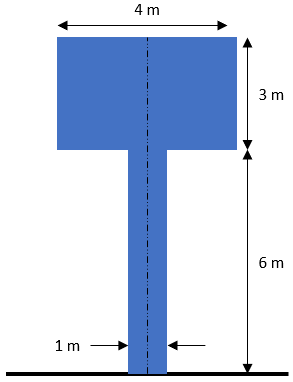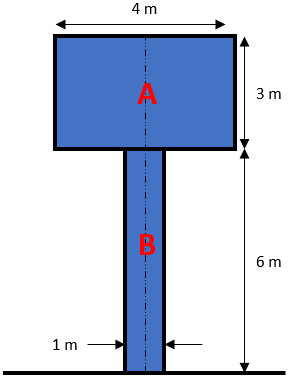## Centroid Area

For the below figure, what is the y-coordinate of the centroid?Hint
Split the object into basic shapes (triangles, rectangles, squares, etc.).Hint 2
The y-component of an area’s centroid is defined as:
$$y_{ac}=\frac{\sum y_n a_n}{A}$$$where $$a_n$$ is the simplified object’s area, $$y_n$$ is the distance from the origin to the middle of the simplified object, and $$A$$ is the total area. The y-component of an area’s centroid is defined as: $$y_{ac}=\frac{\sum y_n a_n}{A}$$$
where $$a_n$$ is the simplified object’s area, $$y_n$$ is the distance from the origin to the middle of the simplified object, and $$A$$ is the total area ( $$\Sigma a_n$$ ). First, let’s split the main object into basic shapes (triangles, rectangles, squares, etc.).To find the y-component of our main object’s centroid, let’s set the ground as the origin:
$$y_{ac}=\frac{(y_A a_A)+(y_B a_B)}{(a_A+a_B)}=\frac{[(\frac{3m}{2}+6m)(4m\cdot 3m)]+[\frac{6m}{2}\cdot(6m\cdot 1m)]}{[(4m\cdot 3m)+(6m\cdot 1m)]}$$$$$=\frac{[(7.5m)(12m^2)+(3m)(6m^2)]}{(12m^2+6m^2)}=\frac{(90m^3+18m^3)}{18m^2}=\frac{108m^3}{18m^2}=6\:m$$$
6 m